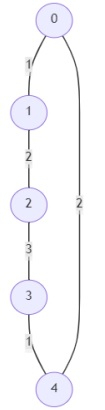# Program to find out if the graph is traversable by everybody in Python

Suppose, we are given a graph that contains n vertices numbered 0 to n - 1. The graph is undirected and each edge has a weight. The graph can have three types of weights and each weight signifies a particular task. There are two people that can traverse the graph, namely Jack and Casey. Jack can traverse the graph if an edge has weight 1, Casey can traverse the graph if it has weight 2, and both can traverse the graph if it has edge weight 3. We have to remove any edges necessary to make the graph traversable for both Jack and Casey. We return the number of edges to remove to make the graph traversable, or we return -1 if it cannot be made traversable.

So, if the input is likeand n =5; then the output will be -1

The graph cannot be made traversable for both by removing an edge. So, the answer is -1.

To solve this, we will follow these steps −

• Define a function find() . This will take val

• if val is not same as root[val], then

• root[val] := find(root[val])

• return root[val]

• Define a function union() . This will take val1, val2

• val1:= find(val1)

• val2 := find(val2)

• if val1 is same as val2, then

• return 0

• root[val1] := val2

• return 1

• res := 0

• edge1 := 0

• edge2 := 0

• root := a new list from range 0 to n + 1

• for each edge (u, v), and its weight w in e, do

• if u is same as 3, then

• if union(v, w) is non-zero, then

• edge1 := edge1 + 1

• edge2 := edge2 + 1

• otherwise,

• res := res + 1

• root0 := root[from index 0 to end]

• for each edge (u, v), and its weight w in e, do

• if u is same as 1, then

• if union(v, w) is non-zero, then

• edge1 := edge1 + 1

• otherwise,

• res := res + 1

• root := root0

• for each edge (u, v), and its weight w in e, do

• if u is same as 2, then

• if union(v, w) is non-zero, then

• edge2 := edge2 + 1

• otherwise,

• res := res + 1

• return res if edge1 is same as edge2 and n - 1

• otherwise, return -1

## Example

Let us see the following implementation to get better understanding

def solve(n, e):
def find(val):
if val != root[val]:
root[val] = find(root[val])
return root[val]
def union(val1, val2):
val1, val2 = find(val1), find(val2)
if val1 == val2: return 0
root[val1] = val2
return 1
res = edge1 = edge2 = 0
root = list(range(n + 1))
for u, v, w in e:
if u == 3:
if union(v, w):
edge1 += 1
edge2 += 1
else:
res += 1
root0 = root[:]
for u, v, w in e:
if u == 1:
if union(v, w):
edge1 += 1
else:
res += 1
root = root0
for u, v, w in e:
if u == 2:
if union(v, w):
edge2 += 1
else:
res += 1
return res if edge1 == edge2 == n - 1 else -1
print(solve(5, [(0,1,1),(1,2,2),(2,3,3),(3,4,1),(4,0,2)]))

## Input

Input:
5, [(0,1,1),(1,2,2),(2,3,3),(3,4,1),(4,0,2)]

## Output

-1